﻿ CMOS-Compatible Linear VCO Using a Single CFOA and Grounded CapacitorsPublications are Open
Access in this journal
Article Versions
Export Article
• Normal Style
• MLA Style
• APA Style
• Chicago Style
Research Article
Open Access Peer-reviewed

### CMOS-Compatible Linear VCO Using a Single CFOA and Grounded Capacitors

D. K. Srivastava, V. K. Singh, R. Senani
American Journal of Electrical and Electronic Engineering. 2017, 5(6), 202-206. DOI: 10.12691/ajeee-5-6-2
Published online: January 16, 2018

### Abstract

A simple CMOS-compatible linear voltage controlled oscillator (VCO) has been presented which employs a single CFOA, four MOSFETs and three grounded capacitors as preferred for IC implementation. In the proposed circuit, variable frequency oscillations are obtained by simultaneous variation of two voltage-controlled-resistors in the circuit which appear in the expression for oscillation frequency in the product form but appear as a ratio in the condition of oscillation (which therefore, remains unaffected). The workability of the proposed VCO has been confirmed by SPICE simulations using an exemplary CMOS CFOA and CMOS VCRs both implementable in 0.18µm CMOS technology.

### 1. Introduction

Analog signal processing/signal generation circuits based on the current feedback operational amplifiers (CFOA) are suitable for high frequency applications as compared to conventional op-amps because of the several advantages offered by them, such as- (i) wider and nearly constant bandwidth independent of gain (for low /medium gains) (ii) higher slew rates (typically 2000V/µsec) and (iii) requirement of a smaller number of external passive components to perform a specified function. A large number of sinusoidal oscillator circuits using CFOAs have been evolved so far, for instance, see 3, 5, 6, 7, 8, 10, 11, 12, 15, 17, 18, 19, 20, 24, 25. While a wide variety of bipolar CFOAs are available as off-the-shelf ICs, on the other hand, several CMOS-based CFOA topologies have also been introduced in the literature, for instance, see 24 and the references cited therein.

The main object of this communication is to introduce a simple voltage-controlled-oscillator (VCO) in which the frequency of oscillation can be varied linearly by simultaneously changing two voltage-controlled-resistances (VCR) in the circuit through an external control voltage VC. These resistors feature in the expression for the condition of oscillation (CO) as a ratio but appear in the frequency of oscillation (FO) as a product. Thus, when these two resistors are replaced by two CMOS VCRs derived by a common external voltage VC, a linear control of the FO results.

### 2. The Proposed Configuration

The CFOA is a four-port active building block which is characterized by the following hybrid matrix: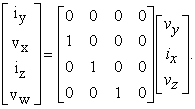(1)

The proposed configuration is shown in Figure 1 which requires only a single CFOA, four MOSFETs and three grounded capacitors with no passive resistors, as preferred for IC implementation 25, 26, 27, 28.

• Figure 1. The proposed Linear VCO

By a straight forward analysis, the condition of oscillation (CO) and the frequency of oscillation (FO) of the circuit are found to be: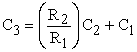(2)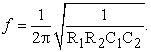(3)

Note that the resistors R2 and R1 appear as ratio in the CO but as product in FO. Thus, if these resistances are varied simultaneously and equally, CO will remain un-affected but oscillation frequency can be varied. Accordingly, these resistor pairs are replaced by two identical CMOS VCRs 1, 16 derived by a common external control voltage VC as shown. The equivalent resistance simulated by each VCR circuit is given by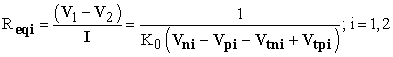(4)

where, K0 is the trans-conductance parameter and VTpi and VTni are the threshold voltages of the ith PMOS and ith NMOS transistors respectively. If we choose R1=R2=R or Req1=Req2=Req with both of these replaced by two identical VCR circuits derived by common control voltages Vni and Vpi, as shown, the eqn. (2) for FO would be modified as: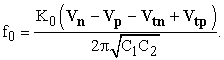(5)

Therefore, it is clear that oscillation frequency has now become a linear function of one of the common control voltages Vni or Vpi (keeping Vpi fixed and Vni variable or vice-versa) and the circuit, thus, realizes a linear VCO.

### 3. Effect of the Parasitic Impedances of the CFOA

Considering the various parasitic impedances of the CFOA, namely, the finite non-zero input resistance Rx at port x, the non-infinite input impedance consisting of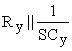at port y and the non-infinite output impedance consisting of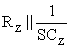at port z, the non-ideal characteristic equation (CE) of the proposed circuit is found to be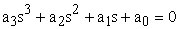(6)

where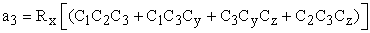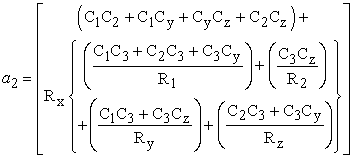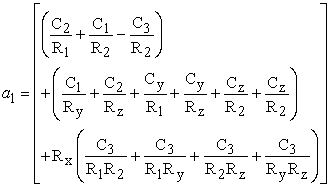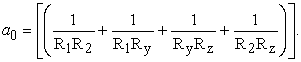From the above, the non-ideal expression for the CO is found to be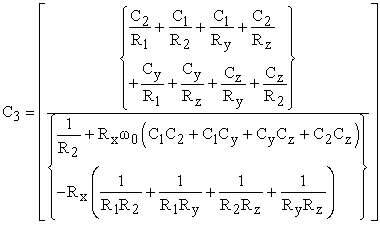(7)

whereas the non-ideal expression for the FO is found to be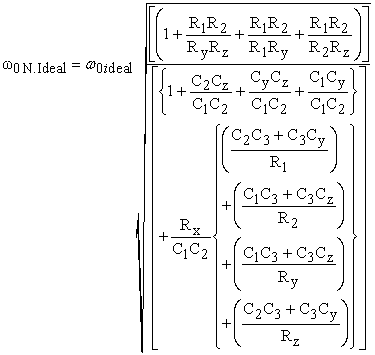(8)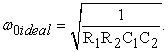(9)

For the designed values of the components as shown in the next section and taking the parasitic components of the CFOA as Rx = 50Ω, Ry = 2MΩ, Cy = 2pF, Rp = 3MΩ,Cp = 4.5pF, it is found that the non-ideal CO is not affected significantly due to the consideration of the various parasitics. On the other hand, in view of the complex formula for the non-ideal FO, it is difficult to draw any qualitative inference from it. However, the simulation results of the oscillator show that their influence also is not very substantial since the frequency obtained from simulations was found to be 7.13MHz which is very close to the value 7.18 MHz calculated from the ideal formula, which, therefore, implies an error of only -0.0069%.

It is worth mentioning that in the literature on CFOA-based circuits, several researchers have conjectured that having a capacitor at x-port may lead to instability in some cases. We now show that this is not true in the present case. In order to check the stability of the circuit, we apply the Routh-Hurwitz criterion for which, the Routh array is constructed as in Table 1.

Therefore, it is clear that there is not any root of the non-ideal characteristic equation in the right half of the s-plane other than the pair of complex conjugate roots which are responsible for generating sinusoidal signals and therefore, are required to be slightly in the right half of the s-plane initially (and eventually movable to the ±jɷ axis). This will happen if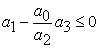(10)

which implies that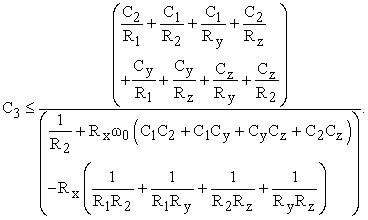(11)

Thus, eqn. (11) should be regarded as the more practicable form of the CO as compared to eqn. (7). It is, therefore, concluded that if the non-ideal CO of the equation (11) is satisfied then there is no other pole which may be located in the right half of the s-plane and thus, the stability of the circuit is also ensured.

### 4. Completely-CMOS Version of the VCO and SPICE Simulation Results

For realizing a linear VCO implementable in CMOS Technology, the CFOA too needs to be realized in CMOS for which a number of alternatives have been proposed in the recent literature. For the present work, we have chosen the CMOS CFOA employed in 19 (which was derived by extracting a CCII+ from the CMOS DDCC of 16 and then appending a CMOS voltage follower to it) which is reproduced here in Figure 2.

• Figure 2. An exemplary CMOS CFOA

For SPICE simulations, the W/L ratios of the MOSFETs used for implementing the CFOA, were chosen as given in Table 2, whereas the aspect ratios of the MOSFETs used to realize the floating VCR circuit were as given in Table 3. Lastly, the model parameters for 0.18μm Technology were taken as given in Table 4.

The SPICE simulation results of the proposed circuit as a linear VCOs are shown in Figure 3 and Figure 4 which show the variation of oscillation frequency with control voltage also.

• Figure 3. Frequency (Simulated)=7.133MHz,THD = 1.83 % with component values C1= 20pF C2=10pF C3=30pF,Vn= 0.75V,Vp= -0.9V
• Figure 4. Variation of oscillation frequency with control voltage

From the SPICE simulations, the circuit was found to perform as predicted by the theory with excellent linearity with respect to the external control voltage. Simulations have shown it to be possible to vary the oscillation frequency from 5.1MHz to 8.4MHz by varying control voltage from 0.3V to 1.4V with % error in the realized frequency lying between 0.0067% to -0.009% only. The power consumed by the circuit was 19.4nW only.

### 5. Comparison with Previously Published Current Conveyors/CFOA-based VCOs

A comparison of the proposed new single-CFOA-based VCO with the previously published Current Conveyors (CC) or CFOA-based VCOs is now in order. It is found that the proposed circuit attains most of the desirable features using only a single CFOA, three grounded capacitors (as preferred for IC implementation 25, 26, 27, 28) along with four MOSFETs only, in contrast to the previously known CC/CFOA-based VCOs of 2, 10, 18, 20, 21, 22, 23, which suffer from the drawbacks of requiring two or more building blocks 2, 9 along with other devices like either a FET and a few additional resistors 18 or eight to twelve MOSFETs 10 or two analog multipliers 20, 21, 22, 23.

### 6. Concluding Remarks

A simple single-CFOA-based three-capacitor-two-resistor oscillator circuit was the main focus of this article which has a tuning law in which two resistors appearing as product in the expression for oscillation frequency appear as a resistor-ratio in the condition of oscillation. By replacing these two resistors by two identical CMOS VCRs derived by a common control voltage, oscillation frequency becomes a linear function of the control voltage, whereas the oscillation condition remains unaffected by this process. The workability of the completely CMOS-compatible version of the circuit obtained by using a CMOS CFOA and CMOS VCRs was verified by SPICE simulations from which the linearity of the variation in the oscillation frequency, with respect to the control voltage, was found to be as predicted by the theory. Lastly, it may be mentioned that the proposed circuit attains most of the desirable features using only a single CFOA, three grounded capacitors (as attractive for IC implementation 25, 26, 27, 28) and four MOSFETs only in contrast to the previously known CC/CFOA-based VCOs of 2, 9, 10, 18, 20, 21, 22, 23.

### References

Published with license by Science and Education Publishing, Copyright © 2017 D. K. Srivastava, V. K. Singh and R. SenaniThis work is licensed under a Creative Commons Attribution 4.0 International License. To view a copy of this license, visit http://creativecommons.org/licenses/by/4.0/